# 22 Results

View
Selected filters: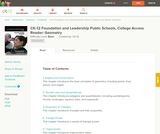Conditions of Use:
Remix and Share
Rating

This book is a "flexed" version of CK-12's Basic Geometry that aligns with College Access Geometry and contains embedded literacy supports. It covers the essentials of geometry for the high school student.

Subject:
Geometry
Material Type:
Textbook
Provider:
CK-12 Foundation
Provider Set:
CK-12 FlexBook
Author:
Fauteux, Michael
Zapata, Rosamaria
05/18/2011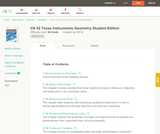Conditions of Use:
Remix and Share
Rating

CK-12 Foundation's Geometry FlexBook is a clear presentation of the essentials of geometry for the high school student. Topics include: Proof, Congruent Triangles, Quadrilaterals, Similarity, Perimeter & Area, Volume, and Transformations.

Subject:
Geometry
Material Type:
Textbook
Provider:
CK-12 Foundation
Provider Set:
CK-12 FlexBook
10/06/2009
Remix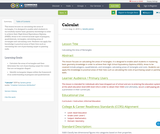Conditions of Use:
No Strings Attached
Rating

This lesson focuses on calculating the areas of rectangles. It is designed to enable adult students to successfully master basic geometry knowledge in order to achieve their High School Equivalency Diploma (HSED). Areas to be covered include types of polygons, quadrilaterals, rectangles; calculating areas of rectangles and calculating costs. Students can apply this knowledge to practical areas of their lives such as calculating the cost of purchasing carpet or painting walls.

Subject:
Mathematics
Material Type:
Interactive
Lesson Plan
Author:
Sandra James
08/08/2019
Remix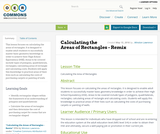Conditions of Use:
No Strings Attached
Rating

This lesson focuses on calculating the areas of rectangles. It is designed to enable adult students to successfully master basic geometry knowledge in order to achieve their High School Equivalency (HSE). Areas to be covered include types of polygons, quadrilaterals, rectangles; calculating areas of rectangle and calculating costs. Students will apply this knowledge to practical areas of their lives such as calculating the costs of purchasing carpets or painting of walls

Subject:
Mathematics
Material Type:
Interactive
Lesson Plan
Author:
Winston Lawrence
08/08/2019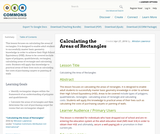Conditions of Use:
No Strings Attached
Rating

This lesson focuses on calculating the areas of rectangles. It is designed to enable adult students to successfully master basic geometry knowledge in order to achieve their High School Equivalency (HSE). Areas to be covered include types of polygons, quadrilaterals, rectangles; calculating areas of rectangle and calculating costs. Students will apply this knowledge to practical areas of their lives such as calculating the costs of purchasing carpets or painting of walls

Subject:
Mathematics
Material Type:
Interactive
Lesson Plan
Author:
Winston Lawrence
05/05/2016
Remix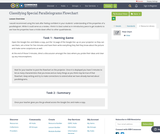Conditions of Use:
Remix and Share
Rating

I would recommned using this task after feeling confident in your students' understanding of the properties of a parallelogram. While it could serve as a review, I think it is best suited as in introductory piece to get students to see how the properties have a trickle down effect to other quadrilaterals.

Subject:
Educational Technology
Mathematics
Material Type:
Lesson Plan
Author:
Mitch Curtis
10/30/2018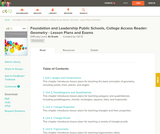Conditions of Use:
Remix and Share
Rating

A structured geometry program teacher edition of daily lesson plans and teacher supports to accompany the College Access Reader: Geometry student edition.

Subject:
Geometry
Material Type:
Textbook
Provider:
CK-12 Foundation
Provider Set:
CK-12 FlexBook
Author:
Fauteux, Michael
Zapata, Rosamaria
05/18/2011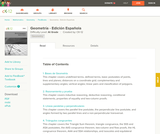Conditions of Use:
Remix and Share
Rating

CK-12 Foundation's Geometry FlexBook is a clear presentation of the essentials of geometry for the high school student. Topics include: Proof, Congruent Triangles, Quadrilaterals, Similarity, Perimeter & Area, Volume, and Transformations.

Subject:
Geometry
Material Type:
Textbook
Provider:
CK-12 Foundation
Provider Set:
CK-12 FlexBook
11/23/2010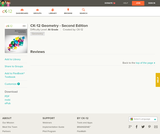Conditions of Use:
Remix and Share
Rating

CK-12's Geometry - Second Edition is a clear presentation of the essentials of geometry for the high school student. Topics include: Proofs, Triangles, Quadrilaterals, Similarity, Perimeter & Area, Volume, and Transformations.

Subject:
Geometry
Material Type:
Textbook
Provider:
CK-12 Foundation
Provider Set:
CK-12 FlexBook
Author:
Dirga, Kathryn
Jordan, Lori
11/16/2010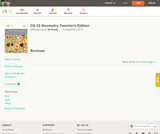Conditions of Use:
Remix and Share
Rating

CK-12 Geometry Teacher's Edition covers tips, common errors, enrichment, differentiated instruction and problem solving for teaching CK-12 Geometry Student Edition. The solution and assessment guides are available upon request.

Subject:
Geometry
Material Type:
Textbook
Provider:
CK-12 Foundation
Provider Set:
CK-12 FlexBook
Author:
Kershaw, Jem
Kramer, Melissa
Zwack, Teresa
06/25/2011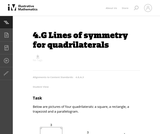Conditions of Use:
No Strings Attached
Rating

This task provides students a chance to experiment with reflections of the plane and their impact on specific types of quadrilaterals. It is both interesting and important that these types of quadrilaterals can be distinguished by their lines of symmetry.

Subject:
Mathematics
Geometry
Material Type:
Activity/Lab
Provider:
Illustrative Mathematics
Provider Set:
Illustrative Mathematics
Author:
Illustrative Mathematics
11/11/2012Rating

Explains the different types of quadrialterals

Subject:
Geometry
Material Type:
Lecture
Provider:
Math Antics
11/23/2016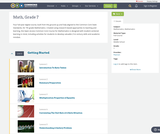Conditions of Use:
Remix and Share
Rating

Four full-year digital course, built from the ground up and fully-aligned to the Common Core State Standards, for 7th grade Mathematics. Created using research-based approaches to teaching and learning, the Open Access Common Core Course for Mathematics is designed with student-centered learning in mind, including activities for students to develop valuable 21st century skills and academic mindset.

Subject:
Mathematics
Material Type:
Full Course
Provider:
Pearson
10/06/2016Conditions of Use:
Remix and Share
Rating

Constructions and Angles

Unit Overview

Type of Unit: Concept

Prior Knowledge

Students should be able to:

Use a protractor and ruler.
Identify different types of triangles and quadrilaterals and their characteristics.

Lesson Flow

After an initial exploratory lesson involving a paper folding activity that gets students thinking in general about angles and figures in a context, the unit is divided into two concept development sections. The first section focuses on types of angles—adjacent, supplementary, complementary, and vertical—and how they are manifested in quadrilaterals. The second section looks at triangles and their properties, including the angle sum, and how this affects other figures.

In the first set of conceptual lessons, students explore different types of angles and where the types of angles appear in quadrilaterals. Students fold paper and observe the angles formed, draw given angles, and explore interactive sketches that test many cases. Students use a protractor and ruler to draw parallelograms with given properties. They explore sketches of parallelograms with specific properties, such as perpendicular diagonals. After concluding the investigation of the angle types, students move on to the next set.

In the second set of conceptual development lessons, students focus on triangles. Students again fold paper to create figures and certain angles, such as complementary angles.

Students draw, using a protractor and ruler, other triangles with given properties. Students then explore triangles with certain known and unknown elements, such as the number of given sides and angles. This process starts with paper folding and drawing and continues with exploration of interactive sketches. Students draw conclusions about which cases allow 0, 1, 2, or an infinite number of triangles. In the course of the exploration, students discover that the sum of the measure of the interior angles of a triangle is 180°. They also learn that the sum of the measures of the interior angles of a quadrilateral is 360°. They explore other polygons to find their angle sum and determine if there is a relationship to angle sum of triangles. The exploration concludes with finding the measure of the interior angles of regular polygons and speculating about how this relates to a circle.

Lastly, students solve equations to find unknown angle measures. Using their previous experience, students find the remaining angle measures in a parallelogram when only one angle measure is given. Students also play a game similar to 20 Questions to identify types of triangles and quadrilaterals. Having completed the remaining lessons, students have a four-day Gallery to explore a variety of problems.

The unit ends with a unit assessment.

Subject:
Mathematics
Geometry
Material Type:
Unit of Study
Provider:
Pearson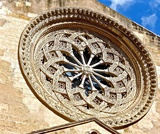Conditions of Use:
Remix and Share
Rating

Lesson OverviewStudents explore different polygons by drawing them, measuring angles, and manipulating interactive sketches to find the angle sum for any polygon. Students also explore the angle measures in regular polygons.Key ConceptsThe angle sum in a triangle is 180°. A quadrilateral can be composed of two triangles, so the angle sum of a quadrilateral is 360°.The number of triangles that compose a polygon is two less than the number of sides (angles). The sum of the interior angles in a polygon with n sides is 180° (n – 2).Goals and Learning ObjectivesFind angle sums in polygons.Generalize to find the angle sum for any polygon.Find interior angle measures for regular polygons.

Subject:
Geometry
Material Type:
Lesson Plan
Provider:
Pearson
09/21/2015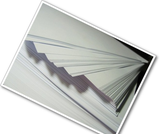Conditions of Use:
Remix and Share
Rating

Gallery OverviewAllow students who have a clear understanding of the content thus far in the unit to work on Gallery problems of their choosing. You can then use this time to provide additional help to students who need review of the unit’s concepts or to assist students who may have fallen behind on work.Problem DescriptionsParallelogram to CubeStudents have a chance to review angle measurements in a parallelogram. Building the cube helps students see the transition from two-dimensional shapes and their relationship to three-dimensional figures.QuadrilateralsStudents investigate the possible quadrilaterals that can be made from any four given side lengths, focusing on those that can’t make a quadrilateral. Students also look at possible parallelograms with two sides given and possible rhombuses with four sides given.DiagonalsStudents further investigate diagonals in quadrilaterals. If the diagonals are perpendicular, is the figure a rhombus?TrapezoidsHow many right angles can a trapezoid have? How many congruent angles or congruent sides can it have? Can its diagonals be perpendicular or congruent? Students investigate possible trapezoids.More AnglesStudents explore three intersecting lines and the combinations of angles.Diagonals and AnglesThe sides of a parallelogram are extended beyond the vertices, and students explore which angles are congruent and which are supplementary. Students also explore the effect diagonals have on interior angles.Exterior AnglesStudents  explore the sum of exterior angles for several polygons and speculate about the results.Angles and SidesStudents explore the relationship between angles and sides in a triangle and discover that the longest side is opposite the largest angle, and the shortest side is opposite the smallest angle (and congruent sides are opposite congruent angles).Ratios and AnglesStudents explore the ratios of the legs of a right triangle to the angles in the triangle. Students see that there is a unique ratio for each angle, and vice versa. This is an informal look at trigonometry.Find the AngleStudents solve equations to find angle measures in polygons.TessellationsStudents explore quadrilateral tessellations and why they tessellate. Students also explore tessellations of pentagons and other polygons.

Subject:
Geometry
Material Type:
Lesson Plan
Provider:
Pearson
09/21/2015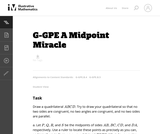Conditions of Use:
No Strings Attached
Rating

This classroom task gives students the opportunity to prove a surprising fact about quadrilaterals: that if we join the midpoints of an arbitrary quadrilateral to form a new quadrilateral, then the new quadrilateral is a parallelogram, even if the original quadrilateral was not.

Subject:
Mathematics
Geometry
Material Type:
Activity/Lab
Provider:
Illustrative Mathematics
Provider Set:
Illustrative Mathematics
Author:
Illustrative Mathematics
05/01/2012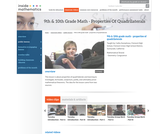Conditions of Use:
Rating

This lesson is about properties of quadrilaterals and learning to investigate, formulate, conjecture, justify, and ultimately prove mathematical theorems. Students will: Analyze characteristics and properties of two- and three-dimensional geometric shapes; develop mathematical arguments about geometric relationships; and apply appropriate techniques, tools, and formulas to determine measurements.Explore relationships among classes of two- and three-dimensional geometric objects, make and test conjectures about them, and solve problems involving them. Employ forms of mathematical reasoning and proof appropriate to the solution of the problem at hand, including deductive and inductive reasoning, making and testing conjectures, and using counter examples and indirect proof. Identify, formulate and confirm conjectures. Establish the validity of geometric conjectures using deduction, prove theorems, and critique arguments made by others. (9th/10th Grade Math)

Subject:
Algebra
Material Type:
Activity/Lab
Lecture
Lesson Plan
Teaching/Learning Strategy
Provider:
Noyce Foundation
Provider Set:
Inside Mathematics
Author:
Humphreys, Cathy
11/30/2011Rating

Subject:
Mathematics
Material Type:
Lesson Plan
Author:
10/02/2016Rating